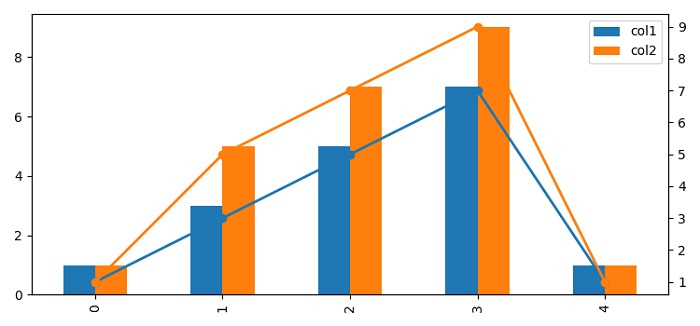# How to align the bar and line in Matplotlib two Y-axes chart?

To align the bar and line in matplotlib two Y-axes chart, we can use twinx() method to create a twin of Axes with a shared X-axis but independent Y-axis.

## Steps

• Set the figure size and adjust the padding between and around the subplots.

• Make a Pandas dataframe with columns 1 and 2.

• Plot the dataframe using plot() method with kind="bar", i.e., class by name.

• Use twinx() method to create a twin of Axes with a shared X-axis but independent Y-axis.

• Plot the axis (Step 3) ticks and dataframe columns values to plot the lines.

• To display the figure, use show() method.

## Example

from matplotlib import pyplot as plt
import pandas as pd

plt.rcParams["figure.figsize"] = [7.50, 3.50]
plt.rcParams["figure.autolayout"] = True

df = pd.DataFrame({"col1": [1, 3, 5, 7, 1], "col2": [1, 5, 7, 9, 1]})
ax = df.plot(kind="bar")
ax2 = ax.twinx()

ax2.plot(ax.get_xticks(),
df[['col1', 'col2']].values,
linestyle='-',
marker='o', linewidth=2.0)

plt.show()

## Output